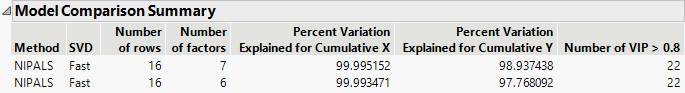Publication date: 11/10/2021

## Model Comparison Summary

The Model Comparison Summary shows summary results for each fitted model.

Figure 6.9 Model Comparison SummaryThe report includes the following summary information:

Method

Shows the analysis method that you specified in the Model Launch control panel.

SVD

Shows the implementation of the SVD algorithm that you specified.

Number of rows

Shows the number of observations used in the training set.

Number of factors

Shows the number of extracted factors.

Percent Variation Explained for Cumulative X

Shows the percent of variation in X that is explained by the model.

Percent Variation Explained for Cumulative Y

Shows the percent of variation in Y that is explained by the model.

Number of VIP>0.8

Shows the number of model effects with VIP (variable importance for projection) values greater than 0.8. The VIP score is a measure of a variable’s importance relative to modeling both X and Y (Wold 1994; Eriksson et al. 2006).